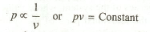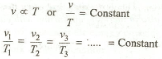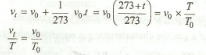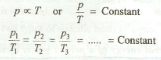# Laws of Perfect Gases

A perfect gas (or an ideal gas) may be defined as a state of a substance, whose evaporation from its liquid state is complete. It may be noted that if its evaporation is partial, the substance is called vapor. A vapor contains some particles of liquid in suspension. The behavior of super-heated vapors is similar to that of a perfect gas.

The physical properties of a gas are controlled by the following three variables :

1. Pressure exerted by the gas,
2. Volume occupied by the gas, and
3. Temperature of the gas.

The behavior of a perfect gas, undergoing any change in these three variables, is governed by the following laws :

1. Boyles law. This law was formulated by Robert Boyle in 1662. It states, "The absolute pressure of a given mass of a perfect gas varies inversely as its volume, when the temperature remains constant." Mathematically,The more useful form of the above equation is :

p1v1 = p2v2 = p3v3 = .. = Constant

where suffixes 1, 2 and 3 refer to different sets of conditions.

2. Charles' law. This law was formulated by a Frenchman Jacques A.C. Charles in about 1787. It may be stated in two different forms :

(i) "The volume of a given mass of a perfect gas varies directly as its absolute temperature, when the absolute pressure remains constant." Mathematically,where suffixes 1, 2 and 3 refer to different sets of conditions.

(ii) "All perfect gases change in volume by 1 / 273 th of its original volume at 0° C for every 1° C change in temperature, when the pressure remains constant."

Let
Vo = Volume of a given mass of gas at 0° C, and
Vt = Volume of the same mass of gas at t° C.

Then, according to the above statement,where

T = Absolute temperature corresponding to t° C.
To = Absolute temperature corresponding to 0° C.

A little consideration will show, that the volume of a gas goes on decreasing by 1/ 273th of its original volume for every 1°C decrease in temperature. It is thus obvious, that at a temperature of — 273° C, the volume of the gas would become zero. The temperature at which the volume of a gas becomes zero is called absolute zero temperature.

3. Gay Lussac law. This law states, "The absolute pressure of a given mass of a perfect gas varies directly as its absolute temperature, when the volume remains constant." Mathematicallywhere suffixes 1, 2 and 3.... refer to different sets of conditions.

Note : In dealing with a perfect gas, the values of pressure and temperature are expressed in absolute units.